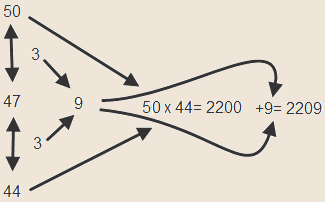Squaring any 2 digit number

This method will help you quickly square any 2 number  fast when none of the previously mentioned tricks can be applied (eg ending in 5, close to 100)

This is an easy method to square a 2 digit number. The Up and Down Method
Let us use 47 for this example.

1)    Seek for the closest number ending in 0 .
In our case we added 3 to reach 50
As we went UP 3 to reach 50 we need to go DOWN 3 from 47 to 44.

2)    Now multiply this two new numbers

50×44=2200
(This should be easy to do given one of the numbers ends in 0)

3)    Add to this number the square of the distance you went UP and DOWN
In our case the distance was 3
The squares distance is 9
2200 +9= 2209 is the answer

The method illustrated in a pictureAnother example where you start by going Down.

Let us use 44 for this example

1)    Seek for the closest number ending in 0 .
In our case we subtracted 4 to reach 40
As we went DOWN 4 to reach 40 we need to go UP 4 from 44 to 48.

2)    Now multiply this two new numbers
40×48=1920

3)    Add to this number the square of the distance you went UP and DOWN
In our case the distance was 4
The squares distance is 16
1920+16= 1936  is the answer